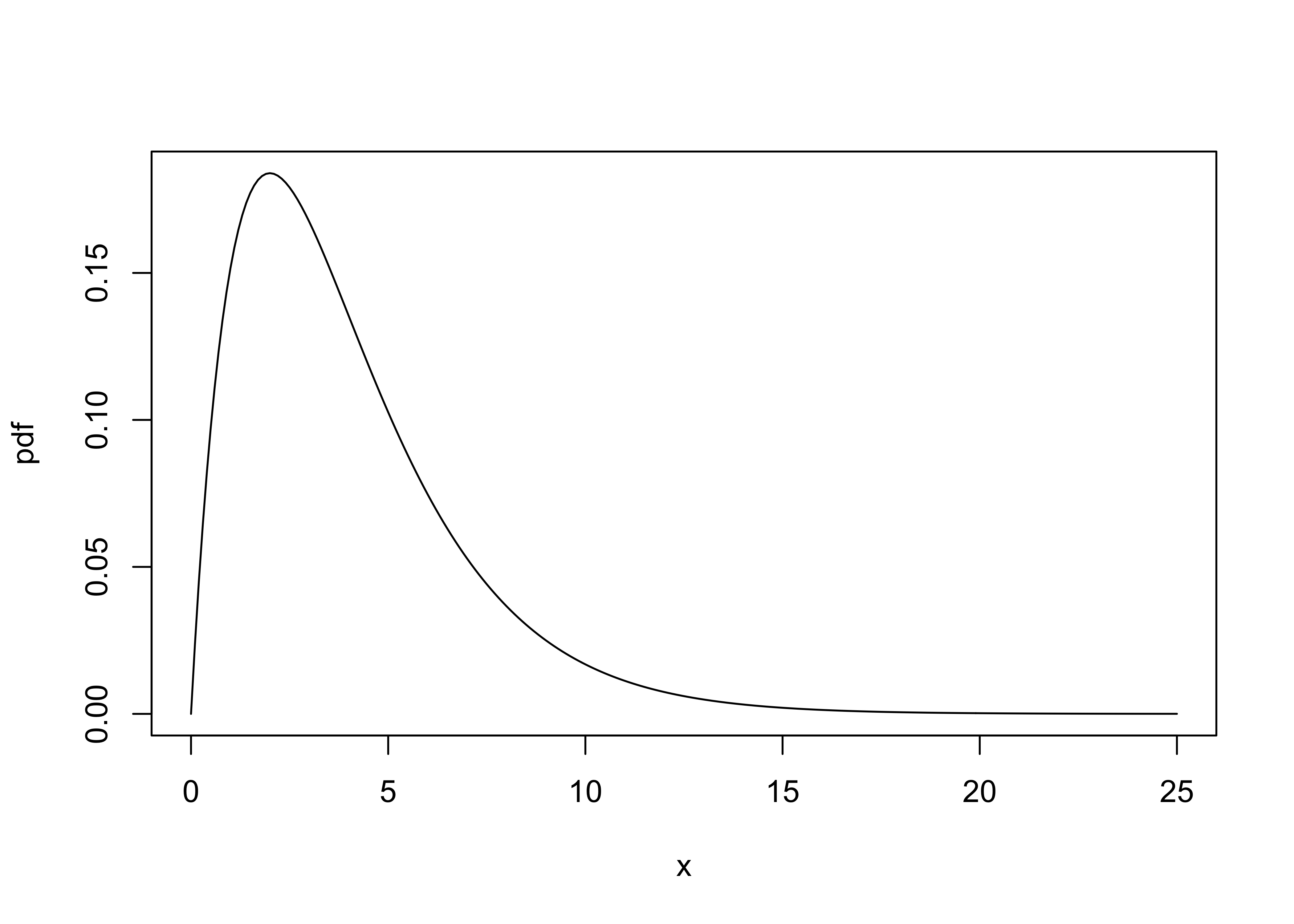NOTE: This page has been revised for Winter 2021, but may undergo further edits.

# 1 Introduction

There are a number of descriptive statistics that, like descriptive plots, provide basic information on the nature of a particular variable or set of variables. A statistic is simply a number that summarizes or represents a set of observations of a particular variable.

Before describing the statistics, it will be helpful to look at the summation operator and

# 2 Univariate descriptive statistics

In general, descriptive statistics–like the univariate descriptive plots–can be classified into three groups, those that measure 1) central tendency or location of a set of numbers, 2) variability or dispersion, and 3) the shape of the distribution. The univariate descriptive statistics can be thought of as companions to the univariate descriptive plots. The best way to develop an idea of what the statistics are summarizing or attempting to convey is to always produce a descriptive plot first.

## 2.1 Measures of Central Tendency

Mode

• definition: the most frequent class interval

Median

• definition: 50th percentile, center point

Mean or Average

Choosing a measure of central tendency

## 2.2 Measures of Variability, Scale or Dispersion

Range

• definition: (maximum value - minimum value)

Interquartile range

• definition: (75th percentile - 25th percentile) (i.e., width of the box in a boxplot)

Variance and standard deviation

Coefficient of variation

## 2.3 Measures of the shapes of distributions

Skewness and kurtosis

# 3 Univariate descriptive statistics – examples

Descriptive statistics can be most easily obtained in R using the summary() function. The summary command is generic in the sense that object or “argument” of the function could be anything. If the argument is a data frame, summary() returns descriptive statistics for each variable, whereas if the argument is a single variable, summary() just returns the descriptive statistics for that variable.

Summarize the scanvote data frame. (Note that it is not necessary to attach the data frame if the whole thing is being summarized.)

# dataframe summary
summary(scanvote) 
##    District              Yes             Pop            Country
##  Length:55          Min.   :25.50   Min.   :   1.50   Length:55
##  Class :character   1st Qu.:43.20   1st Qu.:  10.00   Class :character
##  Mode  :character   Median :48.20   Median :  16.70   Mode  :character
##                     Mean   :47.97   Mean   :  52.02
##                     3rd Qu.:53.80   3rd Qu.:  39.25
##                     Max.   :70.80   Max.   :1016.80

Individual descriptive statistics can be obtained using the following, self-explaining functions:

mean(), median(), max(), min(), range(), var(), sd(), quantile(), fivenum(), length(), which.max(), which.min()

The easiest way to illustrate what these functions do is to apply them to individual variables and see what they produce.

Descriptive statistics for individual groups of observations can be obtained using the tapply() function. For example,

# attach dataframe
attach(scanvote)

# mean of Yes by Country
tapply(Yes, Country, mean)
##      Fin      Nor      Swe
## 52.76667 44.16316 48.57500

The tapply() function applies a particular function, mean() in this case, to groups of observations (specified here by the Country argument), of the variable Yes.

# summary by Country
tapply(Yes, Country, summary)
## $Fin ## Min. 1st Qu. Median Mean 3rd Qu. Max. ## 43.90 47.62 50.10 52.77 55.10 70.80 ## ##$Nor
##    Min. 1st Qu.  Median    Mean 3rd Qu.    Max.
##   25.50   37.20   44.10   44.16   49.55   65.60
##
## $Swe ## Min. 1st Qu. Median Mean 3rd Qu. Max. ## 27.20 45.10 48.90 48.58 53.75 64.80 Detach the scanvote data frame before continuing. # detach the scanvote dataframe detach(scanvote) Here’s a second example, summarizing the variable WidthWS in the Summit Cr. data frame. Note that here the dataframe was not attached prior to executing the code, and so the variables must be indicated by their “full” names (e.g. sumcr$WidthWS):

tapply(sumcr$WidthWS, sumcr$Reach, mean)
##        A        B        C
## 3.981000 2.544783 3.697273

The upstream and downstream grazed reaches (A and C, respecively) have wider stream cross sections than does the exclosure reach (B).

# 4 Bivariate Descriptive Statistics

A frequent goal in data analysis is to efficiently describe or measure the strength of relationships between variables, or to detect associations between factors used to set up a cross tabulation. A related goal may be to determine which variables are related in a predictive sense to a particular response variable, or put another way, to learn how best to predict future values of a response variable. Correlation (and regression analysis), along with measures of association constructed from tables, provide the means for constructing and displaying such relationships.

Bivariate descriptive statistics allow the strength dependence of the relationship displayed in a scatter plot to be efficiently summarized, in much the same way that the univariate descriptive statistics provide efficient summaries of the information evident in univariate plots, but the form of the relationship and possible external influences are best detected using descriptive plots, or by specific analyses like regression.

## 4.1 Correlation and covariance

The correlation coefficient is a simple descriptive statistic that measures the strength of the linear relationship between two interval- or ratio-scale variables (as opposed to categorical, or nominal-scale variables), as might be visualized in a scatter plot. The value of the correlation coefficient, usually symbolized as r, ranges from -1 (for a perfect negative (or inverse) correlation) to +1 for a perfect positive (or direct) correlation.

Data files for these examples (download to the working directory and read in): [cities.csv] [sumcr.csv] [sierra.csv] [orstationc.csv]

## 4.2 Correlation coefficients

[Correlation definition]

[Illustrations of the strength of the correlation]

Produce examples of - a scatter plot matrix (a graphical summary) - the covariance matrix (numerical summary) - the correlation matrix (numerical summary)

# bivariate descriptive statistics with the cities dataframe
attach(cities)
plot(cities[,2:12], pch=16, cex=0.6)  # scatter plot matrix, omit city name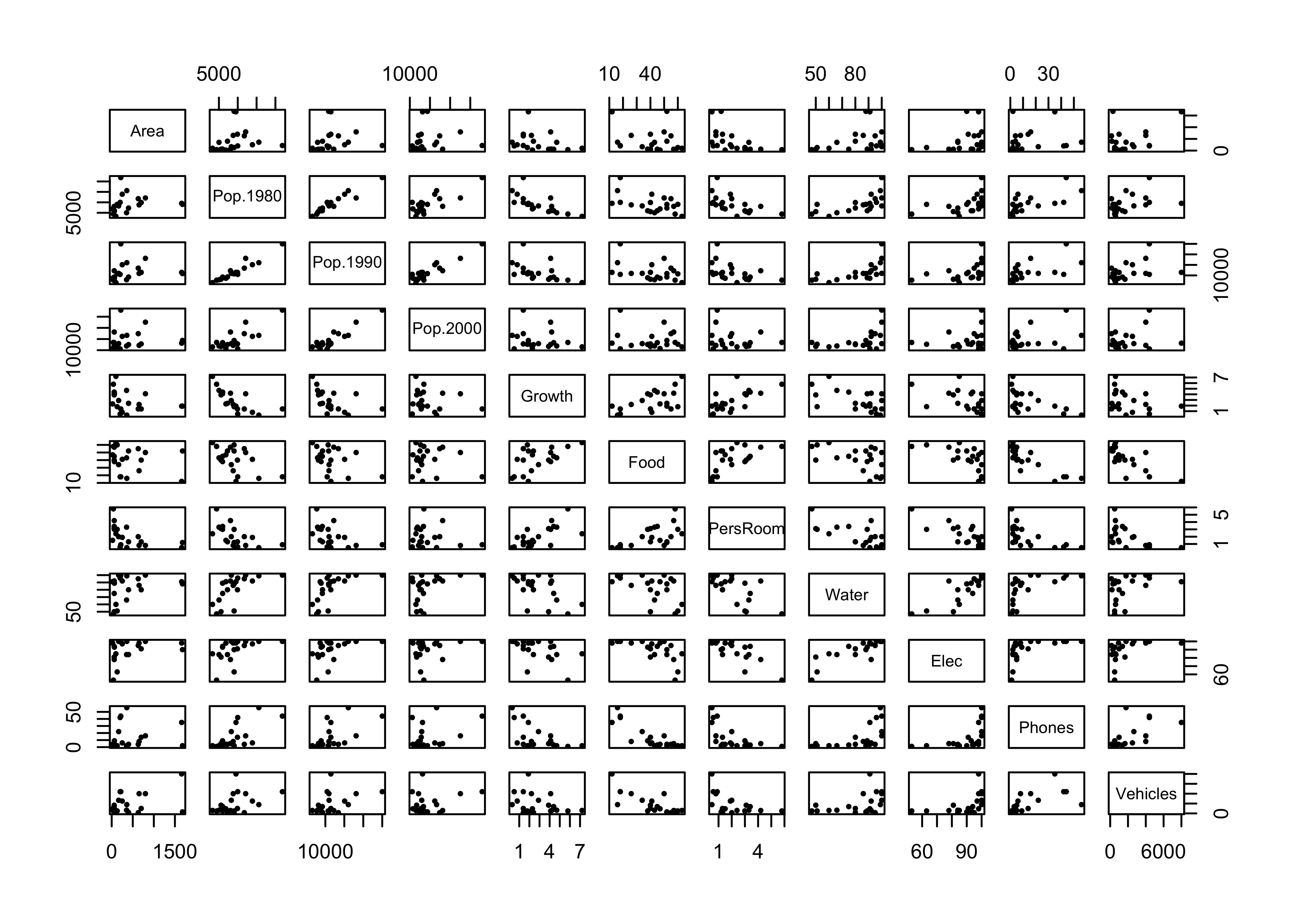cov(cities[,2:12])   # covariance matrix
##                 Area     Pop.1980     Pop.1990     Pop.2000       Growth         Food     PersRoom
## Area     232093.0046   363275.214   300632.844   164425.877  -271.613789  -1694.80643  -346.266284
## Pop.1980 363275.2139 18016775.762 17077452.245 14134672.921 -5342.455944 -34160.30476 -3341.284353
## Pop.1990 300632.8439 17077452.245 17713322.362 16340550.921 -3734.187644 -26529.68810 -2697.674353
## Pop.2000 164425.8767 14134672.921 16340550.921 17680724.429 -1313.215775  -9008.47857 -1096.440770
## Growth     -271.6138    -5342.456    -3734.188    -1313.216     3.293476     15.72238     1.692643
## Food      -1694.8064   -34160.305   -26529.688    -9008.479    15.722381    238.66190    12.915714
## PersRoom   -346.2663    -3341.284    -2697.674    -1096.441     1.692643     12.91571     1.931143
## Water      2473.6629    46038.993    44049.993    33366.307   -17.721429   -136.10714   -16.116429
## Elec       1704.7079    25868.960    23124.476    12426.907   -10.874762   -121.47381   -13.966429
## Phones     1104.0979    47001.560    40624.176    25368.307   -16.119762   -214.12381   -13.541429
## Vehicles 398786.9836  3566388.995  3546252.212  2054176.971 -1172.702601 -23637.23810 -1546.904287
##                Water        Elec      Phones    Vehicles
## Area      2473.66286  1704.70786  1104.09786  398786.984
## Pop.1980 46038.99286 25868.95952 47001.55952 3566388.995
## Pop.1990 44049.99286 23124.47619 40624.17619 3546252.212
## Pop.2000 33366.30714 12426.90714 25368.30714 2054176.971
## Growth     -17.72143   -10.87476   -16.11976   -1172.703
## Food      -136.10714  -121.47381  -214.12381  -23637.238
## PersRoom   -16.11643   -13.96643   -13.54143   -1546.904
## Water      304.71429   183.61429   158.21429   14609.893
## Elec       183.61429   160.44762   107.89762   12780.976
## Phones     158.21429   107.89762   270.34762   20836.276
## Vehicles 14609.89286 12780.97619 20836.27619 4041558.962
cor(cities[,2:12])   # correlation matrix
##                Area   Pop.1980   Pop.1990   Pop.2000     Growth       Food   PersRoom      Water
## Area      1.0000000  0.1776504  0.1482707  0.0811688 -0.3106659 -0.2277181 -0.5172160  0.2941461
## Pop.1980  0.1776504  1.0000000  0.9559486  0.7919490 -0.6935458 -0.5209447 -0.5664580  0.6213565
## Pop.1990  0.1482707  0.9559486  1.0000000  0.9233506 -0.4888987 -0.4080286 -0.4612457  0.5995832
## Pop.2000  0.0811688  0.7919490  0.9233506  1.0000000 -0.1720913 -0.1386787 -0.1876411  0.4545814
## Growth   -0.3106659 -0.6935458 -0.4888987 -0.1720913  1.0000000  0.5607890  0.6711674 -0.5594034
## Food     -0.2277181 -0.5209447 -0.4080286 -0.1386787  0.5607890  1.0000000  0.6016164 -0.5047112
## PersRoom -0.5172160 -0.5664580 -0.4612457 -0.1876411  0.6711674  0.6016164  1.0000000 -0.6643779
## Water     0.2941461  0.6213565  0.5995832  0.4545814 -0.5594034 -0.5047112 -0.6643779  1.0000000
## Elec      0.2793523  0.4811426  0.4337657  0.2333170 -0.4730705 -0.6207611 -0.7934353  0.8304116
## Phones    0.1393848  0.6734610  0.5870475  0.3669274 -0.5402193 -0.8429700 -0.5926469  0.5512366
## Vehicles  0.4117518  0.4179417  0.4191265  0.2430040 -0.3214298 -0.7610807 -0.5537093  0.4163189
##                Elec     Phones   Vehicles
## Area      0.2793523  0.1393848  0.4117518
## Pop.1980  0.4811426  0.6734610  0.4179417
## Pop.1990  0.4337657  0.5870475  0.4191265
## Pop.2000  0.2333170  0.3669274  0.2430040
## Growth   -0.4730705 -0.5402193 -0.3214298
## Food     -0.6207611 -0.8429700 -0.7610807
## PersRoom -0.7934353 -0.5926469 -0.5537093
## Water     0.8304116  0.5512366  0.4163189
## Elec      1.0000000  0.5180646  0.5019066
## Phones    0.5180646  1.0000000  0.6303538
## Vehicles  0.5019066  0.6303538  1.0000000
detach(cities)

## 4.3 Correlation coefficients only measure linear relationships

An important issue in the calculation and interpretation of correlations and covariances is that they only measure or describe linear relationships. This can be illustrated by the relationship between water surface width and downstream distance at Summit Cr.:

# scatter plot with smooth
attach(sumcr)
plot(CumLen, WidthWS)
lines(lowess(CumLen, WidthWS), col="blue", lwd=2)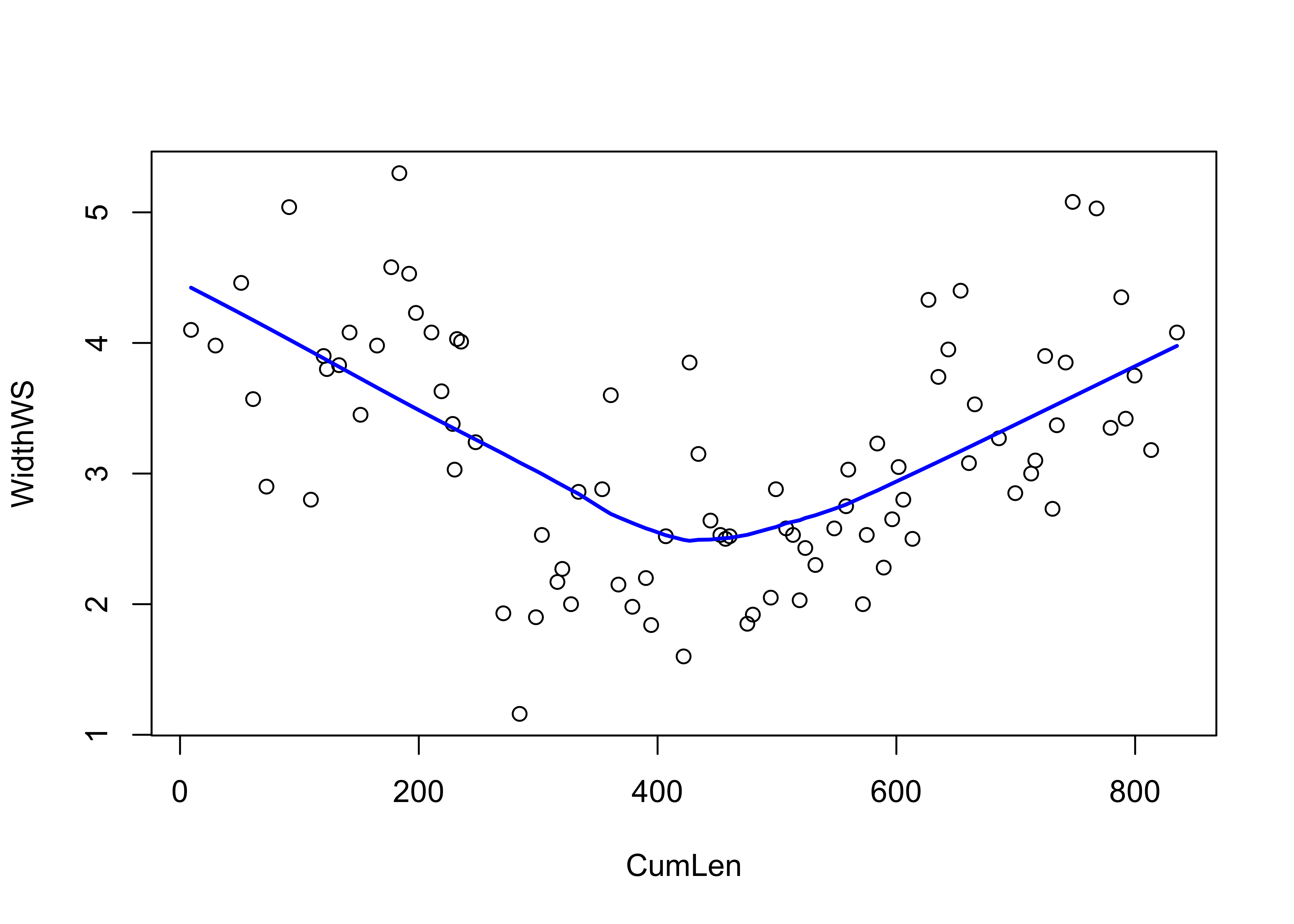The relationship is obviously non-linear, but strong (reflecting the among-reach differences in WidthWS seen earlier). What about the correlation?

cor(CumLen, WidthWS)
##  -0.0959915
detach(sumcr)

Does the correlation coefficient make any sense here?

# 5 The X2 (Chi-square) measure of association (for categorical data)

Categorical data are data that take on discreet values corresponding to the particular class interval that observations of ordinal-, interval-, or ratio-scale variables fall in or the particular group membership of nominal-scale variables. Before applying a particular descriptive statistic, it’s always good to plot the data.

## 5.1 Descriptive plots for categorical data–mosaic plots

Categorical or group-membership data (“factors” in R) are often summarized in tables, the cells of which indicate absolute or relative frequencies of different combinations of the levels of the factors. There are several approaches for visualizing the contents of a table.

First, summarize the data in a table (sometimes called a “cross-tab” or “cross-tabulation” table):

# descriptive plots for categorical data
ReachHU_table <- table(Reach, HU)   # tabluate Reach and HU data
ReachHU_table
##      HU
## Reach  G  P  R
##     A  6  4 10
##     B 12 13 21
##     C  9  5  8

Next, produce several summary plots based on the table:

dotchart(ReachHU_table)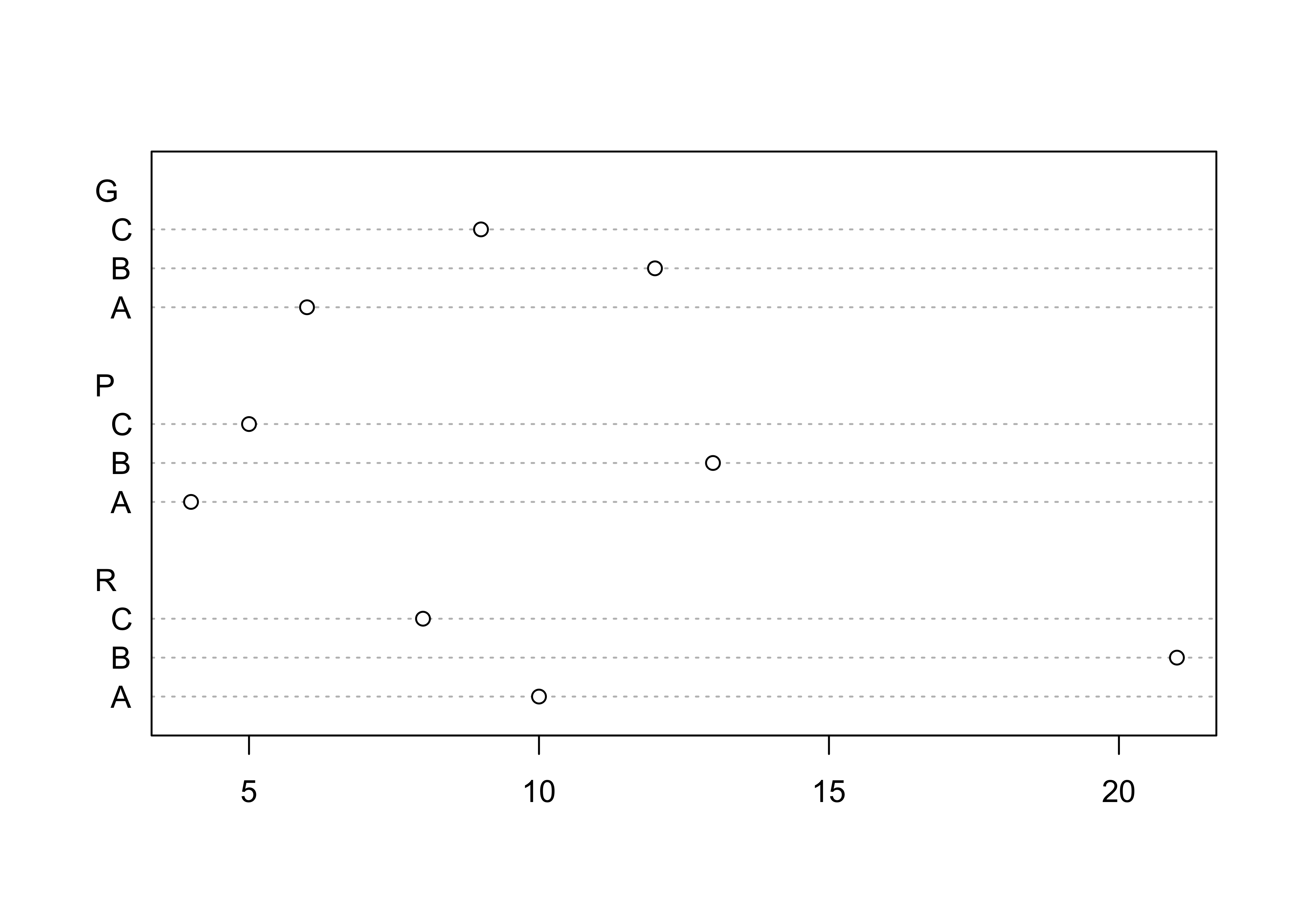barplot(ReachHU_table)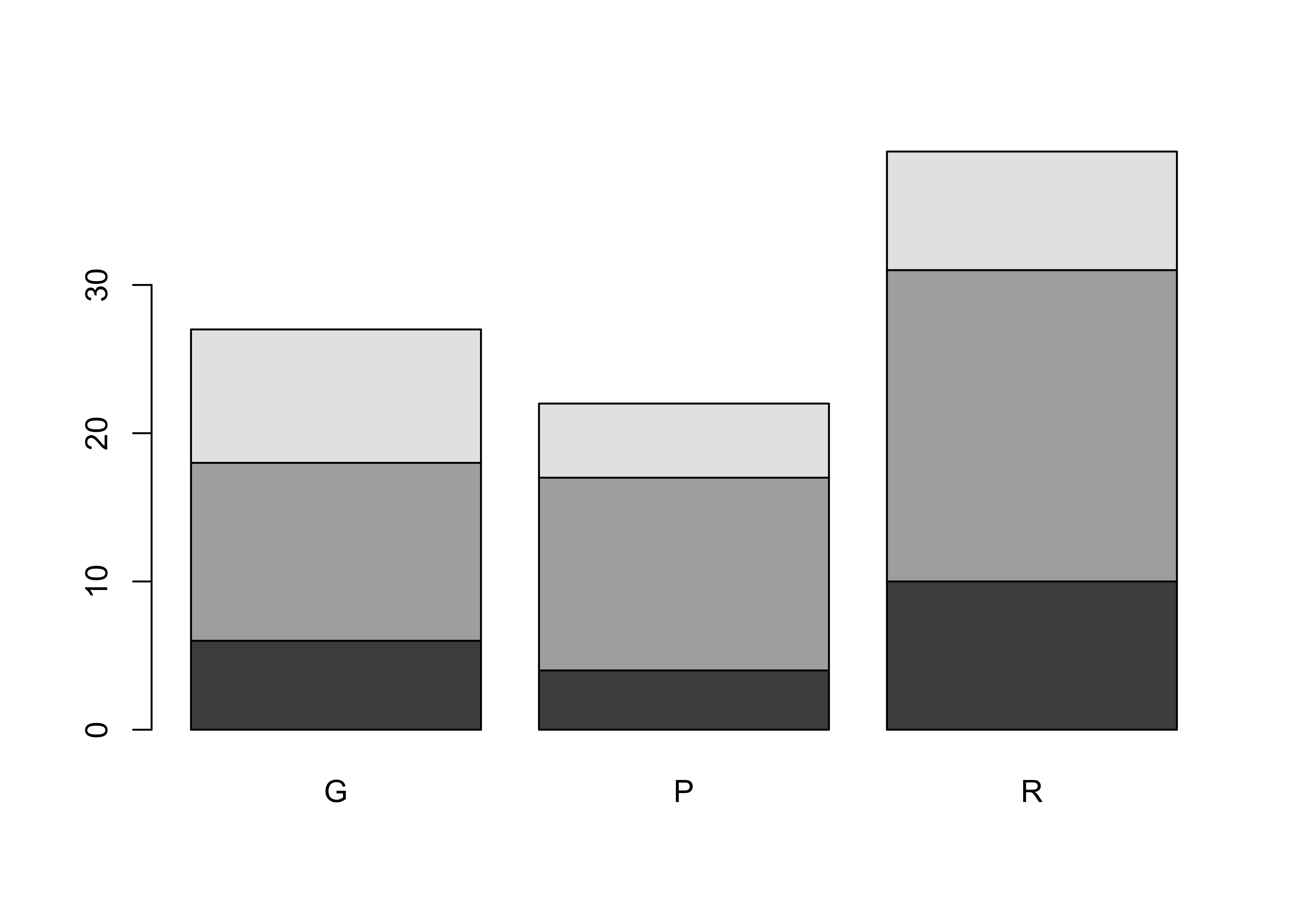mosaicplot(ReachHU_table, color=T)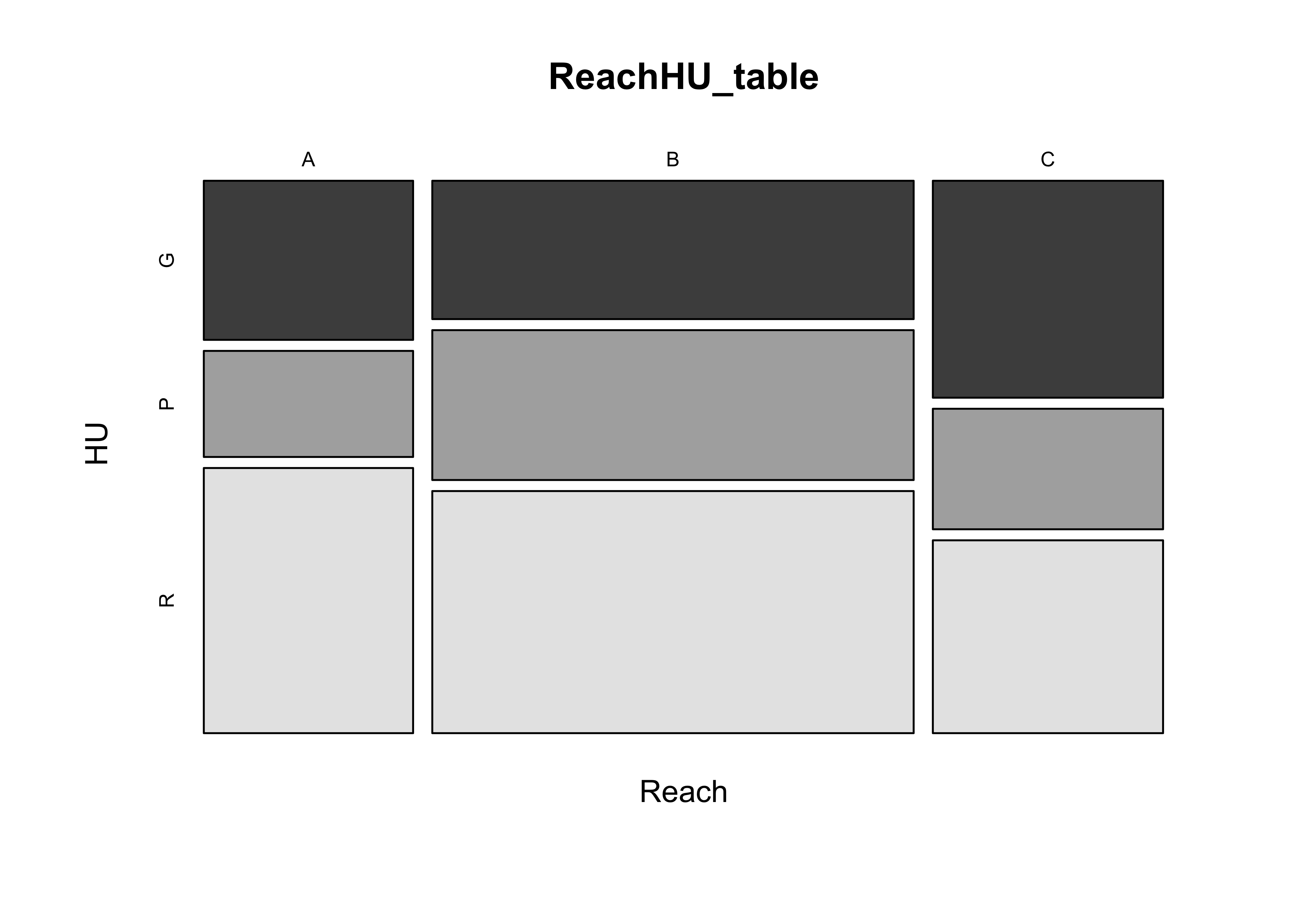## 5.2 The Chi-square statistic

The X2 statistic measures the strength of association between two categorical variables (nominal- or ordinal-scale variables, summarized by a cross-tabulation, a table that shows the frequency of occurrence of observations with particular combinations of the levels of two (or more) variables.

[Chi-squared definition]

Calculate the X2 statistic for the ReachHU table.

# Chi-squared statistic
ReachHU_table <- table(Reach,HU)
ReachHU_table
##      HU
## Reach  G  P  R
##     A  6  4 10
##     B 12 13 21
##     C  9  5  8
chisq.test(ReachHU_table)
##
##  Pearson's Chi-squared test
##
## data:  ReachHU_table
## X-squared = 1.9889, df = 4, p-value = 0.7378

The p-value reported here provides a way of inferring whether or not there is a relationship between the row and column variables in the table, and will be explained more later. In practice the value here is rather large, which provides support for rejecting the notion that there is a relationship between Reach and HU, and so we might conclude instead that they are “independent”.

detach(sumcr)

To further illustrate the application of the X2 test, the Sierra Nevada reconstructed climate data and the Oregon climate-station data can be converted to categorical (ordinal-scale) data, and the following scripts employed

# crosstab & Chi-square -- Sierra Nevada TSum and PWin groups
attach(sierra)
plot(PWin, TSum)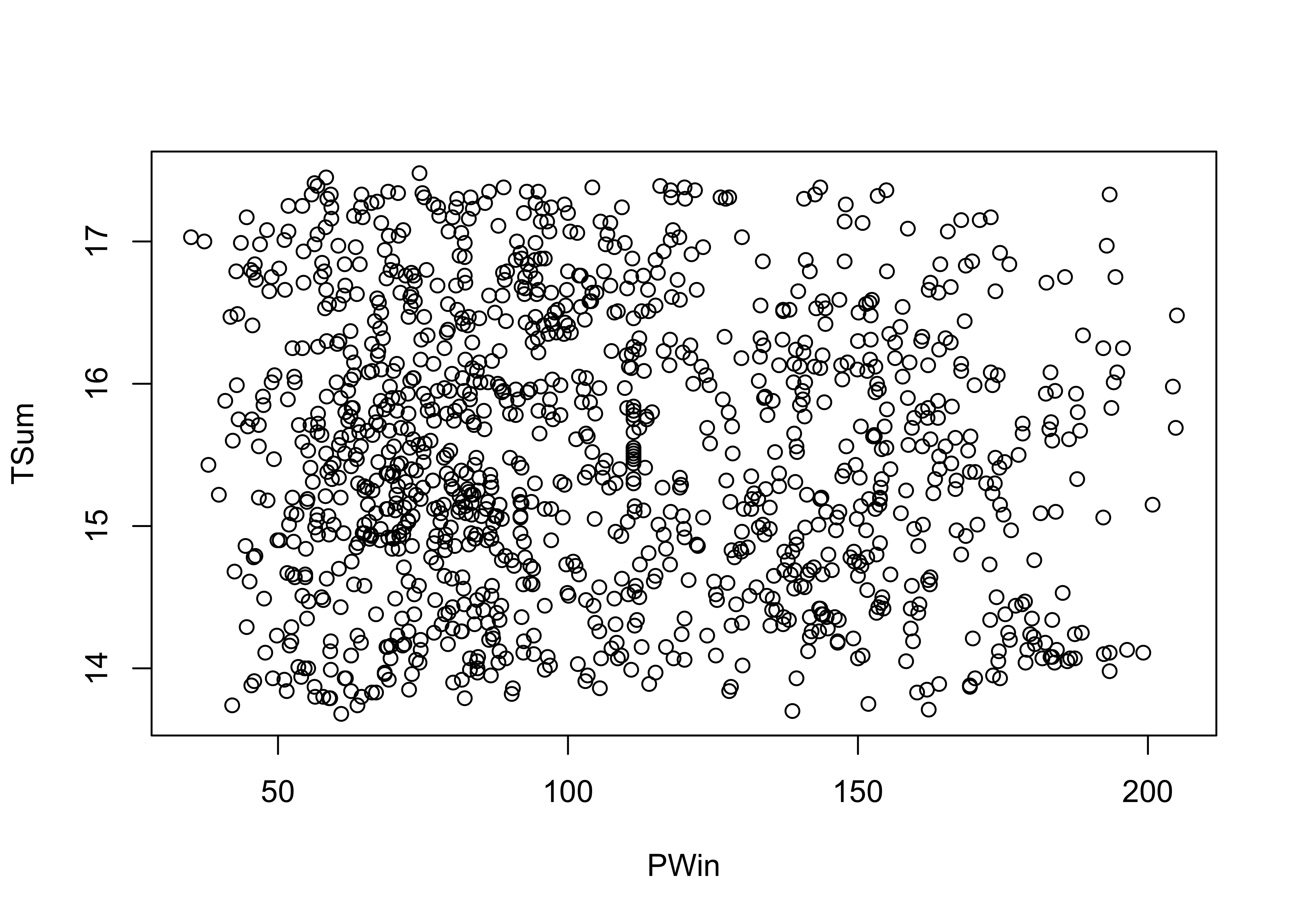PWin_group <- cut(PWin, 3)
TSum_group <- cut(TSum, 3)
TSumPWin_table <- table(TSum_group, PWin_group)
TSumPWin_table
##              PWin_group
## TSum_group    (34.8,91.7] (91.7,148] (148,205]
##   (13.7,14.9]         166        130        79
##   (14.9,16.2]         239        152       101
##   (16.2,17.5]         142        135        45
chisq.test(TSumPWin_table)
##
##  Pearson's Chi-squared test
##
## data:  TSumPWin_table
## X-squared = 13.733, df = 4, p-value = 0.008198
detach(sierra)

The X2 test here yields a small p-value, suggesting that there is a relationship between the two variables.

# crosstab & Chi-square -- Oregon station elevation and tann
attach(orstationc)
## The following objects are masked _by_ .GlobalEnv:
##
##     lat, lon
plot(elev, tann)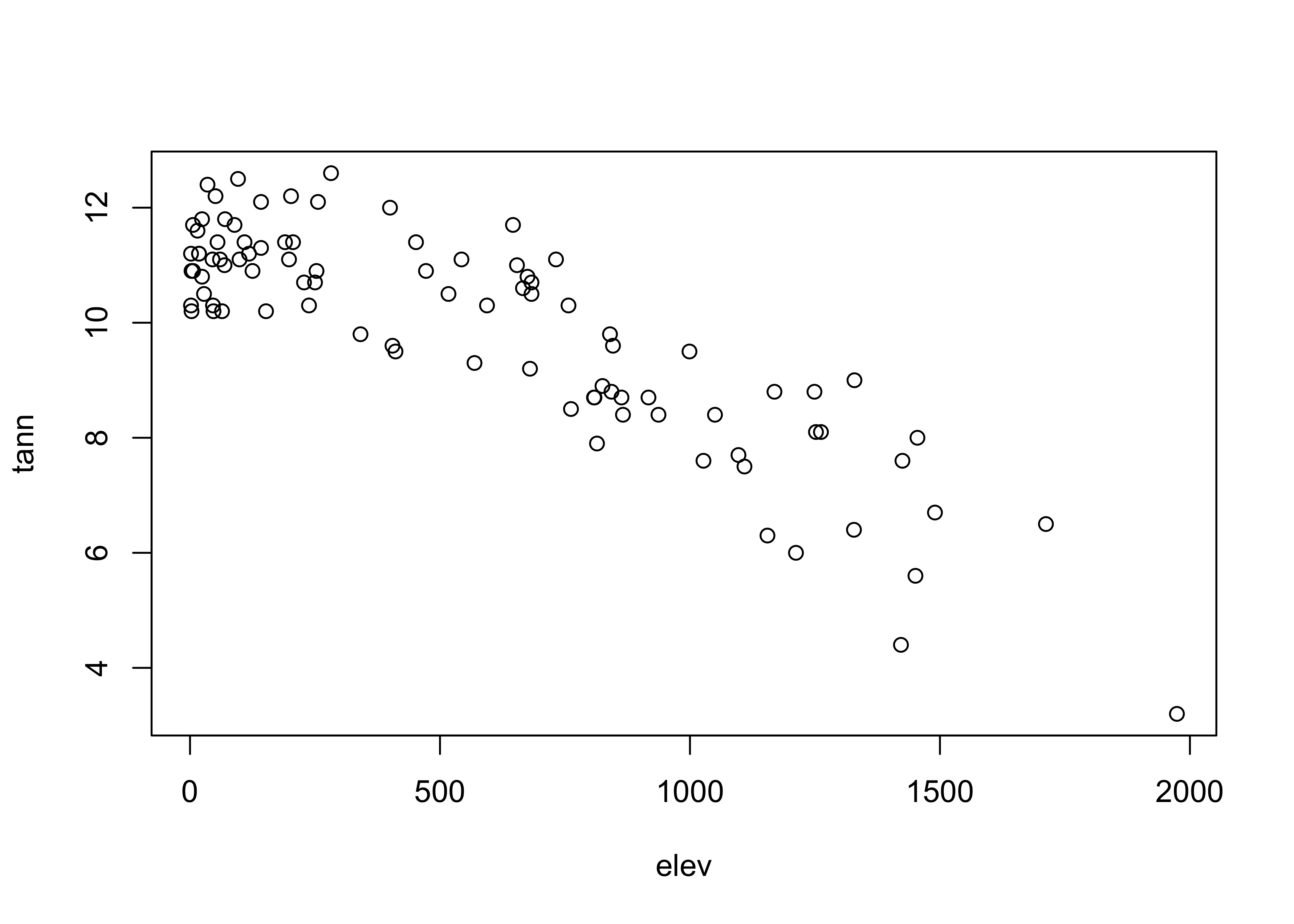elev_group <- cut(elev, 3)
tann_group <- cut(tann, 3)
elevtann_table <- table(elev_group, tann_group)
elevtann_table
##                      tann_group
## elev_group            (3.19,6.33] (6.33,9.47] (9.47,12.6]
##   (0.028,659]                   0           1          52
##   (659,1.32e+03]                2          19           9
##   (1.32e+03,1.98e+03]           3           6           0
chisq.test(elevtann_table)
## Warning in chisq.test(elevtann_table): Chi-squared approximation may be incorrect
##
##  Pearson's Chi-squared test
##
## data:  elevtann_table
## X-squared = 66.672, df = 4, p-value = 1.143e-13

The X2 test here yields a small p-value, again suggesting that there is a relationship between the two variables.

## 5.3 The Chi-square distribution

Quick look at the appropriate Chi-square distribution:

x <- seq(0, 25, by = .1)
pdf <- dchisq(x, 4)
plot(pdf ~ x, type="l")